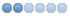• bqgray
• Offline
• Junior Member
•14 Oct 2009 20:42 #976 by bqgray
So,

I guess I'm in the process of becoming less of a newbie.

I've written a piece of g-code (o-code?). The purpose of this code is to put a surface on a given area. I have been hoping to make it really accessable, so that the primary program variables will be easy to alter for any user.

I wrote the code, and now I would like to load it into my machine, but when I try the EMC2 Axis interface simply hangs. It is a very simple piece of code (at least from a programming perspective)

What can I do to make this load/work?

Thanks,

Bill
```(Process Variables)
#11 = 5  		(X Max)
#12 = 4			(X Min)
#19 = #11		(X Current Location - Start at Max)

#21 = 4  		(Y Max)
#22 = 3			(Y Min)
#23 = 0.2		(Y Step)
#28 = [0 - #23]		(Y Step with Direction - Start out Decrementing)
#29 = #21       	(Y Current Location - Start at Max)

#31 = 1.2		(Z Max)
#32 = 1			(Z Min)
#33 = 0.05		(Z Step)
#39 = #31		(Z Current Location - Start at Max)

g0 x#19 y#29 z#39  (Set Starting Point)

o1 while [ #39 GT #32 ]  (Z loop steps down)

g0 z#39

o5 if [#28 LT 0]  (If step direction is down, step down. Else step up)

o2 while [#29 GT #22] (Y loop steps down)

g0 y#29

o3 if [#19 EQ #11 ]  (X pass min to max to min)
#19 = #12
o3 else
#19 = #11
o3 endif

g0 x#19

#29 = [#29 + #23]  (Decrement Y)

o2 endwhile

o5 else

o6 while [#29 LT #21] (Y loop steps up)

g0 y#29

o7 if [#19 EQ #11 ]  (X pass min to max to min)
#19 = #12
o7 else
#19 = #11
o7 endif

g0 x#19

#29 = [#29 + #23]  (Increment Y)

o6 endwhile
o5 endif

#23 = [0 - #23] 	(Reverse Y Step Direction)

#39 = [#39 - #33]  	(Decrement Z)

o1 endwhile
M2```

• bqgray
• Offline
• Junior Member
•15 Oct 2009 17:11 - 15 Oct 2009 17:12 #980 by bqgray
I've done some more research, and it would seem that Axis is not really able to handle the "while" loop at all?

Below is my new test code. If I simple remove the while statement, things load up fine. With the while statement Axis hangs. Seems that there might be a bug here? Or am I missing something?

Thanks,

B
```(Process Variables)
#11 = 5  		(X Max)
#12 = 4			(X Min)
#19 = #11		(X Current Location - Start at Max)

#21 = 4  		(Y Max)
#22 = 3			(Y Min)
#23 = 0.2		(Y Step)
#29 = #21       	(Y Current Location - Start at Max)

#31 = 1.2		(Z Max)
#32 = 1			(Z Min)
#33 = 0.05		(Z Step)
#39 = #31		(Z Current Location - Start at Max)

g0 x#19 y#29 z#39  (Set Starting Point)

o2 while [#29 GT #22] (Y loop steps down)

g0 y#29

#29 = [#29 - #23]  (Decrement Y)

o2 endwhile

m2```
Last edit: 15 Oct 2009 17:12 by bqgray.

• bqgray
• Offline
• Junior Member
•15 Oct 2009 23:45 #981 by bqgray
Well, a little more beating my head against this I seem to have something working.

All I can say is that the code seems to be real picky about capitalization and numbers.

Using capital O and three digit O numbers such as O123 not o2 appears to make the whole show work.

Pretty weird that that doesn't through an error and instead just makes the system hang, but whatever.

Thanks,

Bill